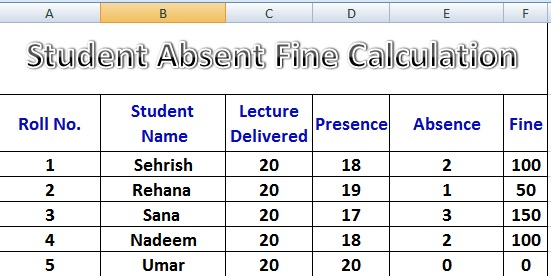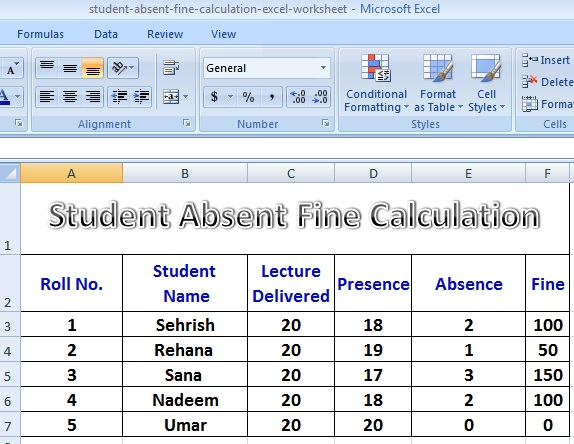# Excel Worksheet Student Absence Fine Calculation Formulas

Today, we will discuss how to create an Excel Worksheet that helps calculate Students Absence Fine Calculation on basis of Total Lectures Delivered and Total Lectures Attended (Presence) of the student.Excel Worksheet Student Absence Fine Calculation Formulas

### Steps To Prepare an Excel Worksheet for Student Absence Fine Calculation

1. Start MS Excel with a blank workbook option.
2. Type sample data in first Excel worksheet as shown in the given image.
3. Type formula for calculation of number of absence of a student.
Place cursor in Cell with reference E3.